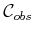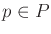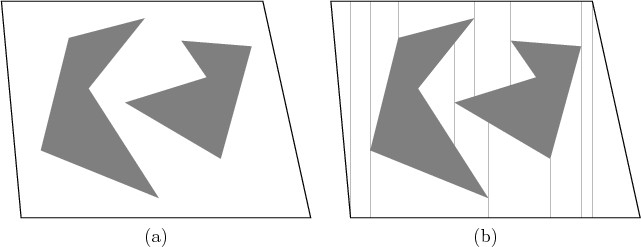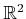#### Defining the vertical decomposition

We next present an algorithm that constructs a vertical cell decomposition , which partitionsinto a finite collection of 2-cells and 1-cells. Each 2-cell is either a trapezoid that has vertical sides or a triangle (which is a degenerate trapezoid). For this reason, the method is sometimes called trapezoidal decomposition. The decomposition is defined as follows. Letdenote the set of vertices used to define. At every, try to extend rays upward and downward through, untilis hit. There are four possible cases, as shown in Figure 6.2, depending on whether or not it is possible to extend in each of the two directions. Ifis partitioned according to these rays, then a vertical decomposition results. Extending these rays for the example in Figure 6.3a leads to the decomposition ofshown in Figure 6.3b. Note that only trapezoids and triangles are obtained for the 2-cells in.Every 1-cell is a vertical segment that serves as the border between two 2-cells. We must ensure that the topology ofis correctly represented. Recall thatwas defined to be an open set. Every 2-cell is actually defined to be an open set in; thus, it is the interior of a trapezoid or triangle. The 1-cells are the interiors of segments. It is tempting to make 0-cells, which correspond to the endpoints of segments, but these are not allowed because they lie in.

Steven M LaValle 2020-08-14